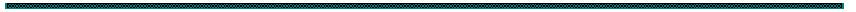Direct PZT Fluid Flow Control Feasibility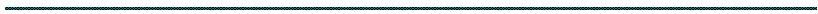1. Incompressible flow in the round pipe.a). In laminar flow , fluid particles move along straight, parallel paths in layers or laminae. Magnitudes of velocities of adjacent laminae are not the same.
Laminar flow is governed by the law relating shear stress to rate of angular
deformation, i.e., the product of viscosity of fluid and velocity gradient or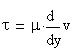In laminar flow, t he viscosity of the fluid is dominant and thus suppresses any tendency to turbulent conditions.
Flow became turbulent, when velocity has reached value of the critical velocity (is the velocity below which all turbulence is damped out by the viscosity of the fluid). It is found that the upper limit of laminar flow of practical interest is represented by a Reynolds number of about 2000.
Velocity distribution at a cross section will follow a parabolic law of variation for laminar flow. Maximum velocity is at the center and is twice the average velocity. The equation of the velocity profile for laminar flow can be expressed asfor circle pipe velocity distribution is parabolic.
or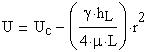where hL is the lost head:Volumetric flow via round/circle pipe is:using finite element approximation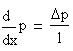and equation for velocity distribution obtain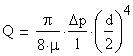famous Poiseuille equation.
In this case average velocity is:and maximum velocity (r=0) is:B. Incompressible flow in the Rectangular pipe (duct).Velocity distribution at a cross section will follow a parabolic law of variation for laminar flow. Maximum velocity is at the center and is twice the average velocity. The equation of the velocity profile for laminar flow can be expressed as
Duct with dimension: 2a x 2b and b - is a gap.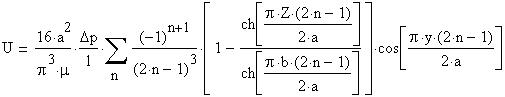for rectangular pipe.where: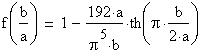For laminar flow between two flat plates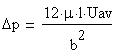where b is a gap
Friction factor f can be derived mathematically for laminar flow, but no simple mathematical relation for the variation of f with Reynolds number is available for turbulent flow. Furthermore, Nikuradse and others found that the relative roughness of a pipe (ratio of size of surface imperfections e to inside diameter of the pipe) affects the value of f also.
(a) Thus, for laminar flow in all pipes for all fluids, the value of f isRe has a practical maximum
value of 2000 for laminar flow.
A. Incompressible flow in the round pipe.
Flow characterization without forcing from DPZTFFC device (only viscosity changes which allowed change
character of turbulent flow) for steady, incompressible flow in the round pipe only.
Laminar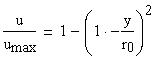Turbulent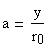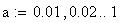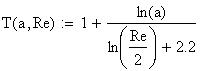Average velocity for laminar flow is 0.5 of maximum velocity in the center-line (parabolic profile).
Average velocity for turbulent flow is a Re number dependent and for Re=5,000 equal 0.77 and for Re=3*10^6 equal 0.87 of maximum velocity in center-line ( logarithmic profile).
DPZTFFC device authority estimation: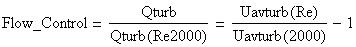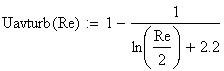In this case (round pipe flow) control authority would be limited by 4-5%.
However, in assumption that the DPZTFFC will change interaction between flow and wall surface we can change the velocity to make flow turbulent earlier. For example, for pipe with 10 mm dia. and water as media Re~10^4*V and critical velocity is 0.2 m/sec. Presumably the DPZTFFC could make the critical velocity smaller. In this case ( laminar / turbulent flow transfer) control authority would be 50-80% of flow.
B. Incompressible flow between flat plates.For laminar boundary layer, if Re high enough: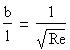where : b - is boundary layer thickness;
l - character length (plate f.e.)
For our case Re~10^4...10^5 and this number give us boundary layer with gap b~(0.003...0.010)*l
If device will used PZT plate with l=100 mm we will capable to control gap b~(0.3...1) mm.
Let's assume b=0.5 mm for l=100 mm. Let's assume also 2*a=100 mm.
According to equation ( ) for laminar flow in rectangular duct with dimension: 2a x 2b (b - is a gap) the average velocity could be calculated as:
Device would be capable for maximum flow: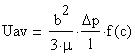where: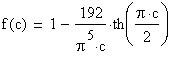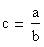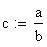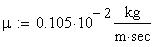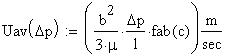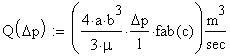We can see device with good possibility for get authority on flow and with reasonable flow characteristics.
With drop of the pressure less then 1 atm volumetric flow rate would be close to 30 m3 per hour.
Developed by Roman N Tunkel,
Research Engineer, Ph.D.,
tunkelr@gmail.com